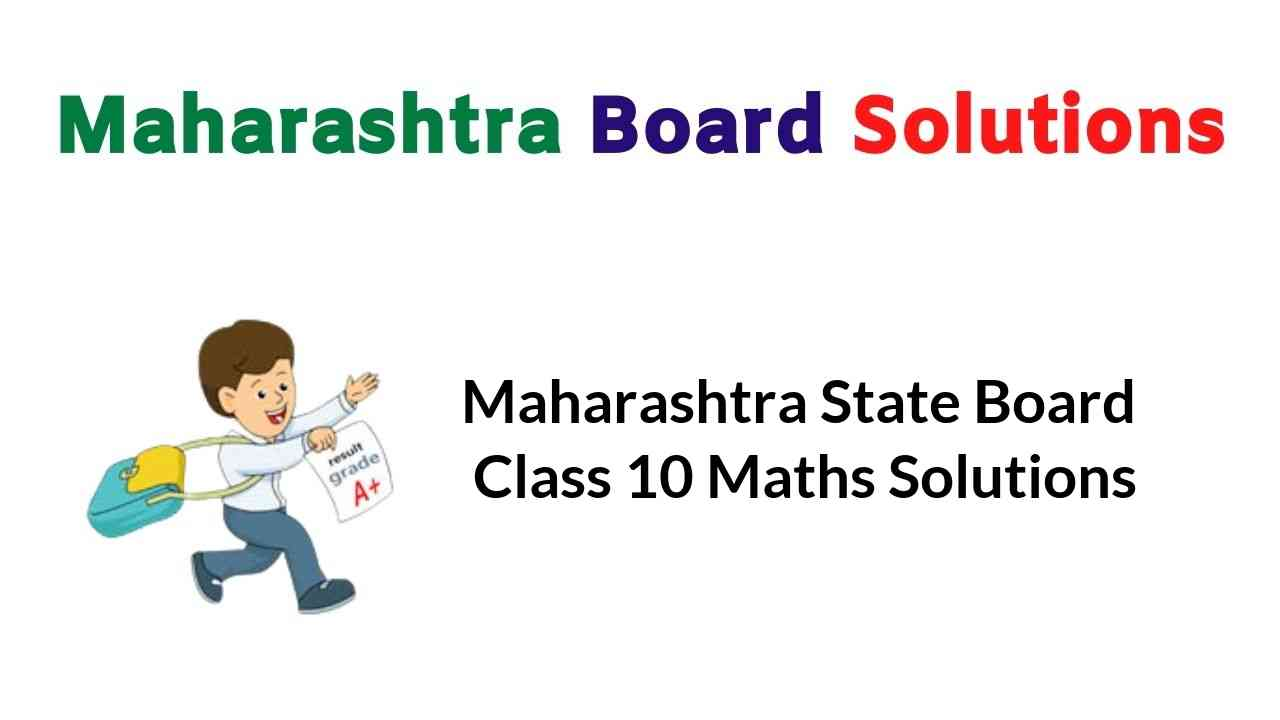Expert Teachers has created Maharashtra State Board Class 10 Maths Solutions Algebra Geometry Part 1 & 2 Pdf. You can also Download Solution of 10th Class Maths Maharashtra Board Pdf to help you to revise complete Syllabus and score more marks in your examinations.

## Balbharati Maharashtra State Board 10th Std Maths Textbook Solutions Answers Digest Part 1 & 2

### Maharashtra State Board Class 10 Maths Solutions Algebra Pdf Part 1

Problem Set 1 Geometry 10th Maharashtra Board Chapter 1 Linear Equations in Two Variables

Maharashtra State Board Class 10 Maths Solutions Chapter 2 Quadratic Equations

Problem Set 3 Algebra Class 10 Chapter 3 Arithmetic Progression

Financial Planning Class 10 Pdf Chapter 4

Practice Set 5.1 Geometry Class 10 Chapter 5 Probability

Problem Set 6 Algebra Class 10 Chapter 6 Statistics

### Maharashtra State Board Class 10 Maths Solutions Geometry Pdf Part 2

Maharashtra Board Class 10 Maths Chapter 1 Similarity

Maharashtra Board Class 10 Maths Chapter 2 Pythagoras Theorem

Maharashtra Board Class 10 Maths Chapter 3 Circle

Problem Set 6 Geometry Class 10 Chapter 4 Geometric Constructions

Maharashtra State Board Class 10 Maths Solutions Geometry Chapter 5 Co-ordinate Geometry

Trigonometry 10th Class Problem Set 6 Maharashtra Board Class 10 Maths Chapter 6

Problem Set 7 Geometry 10th Maharastra Board Chapter 7 Mensuration

Maharashtra State Board Class 10 Textbook Solutions